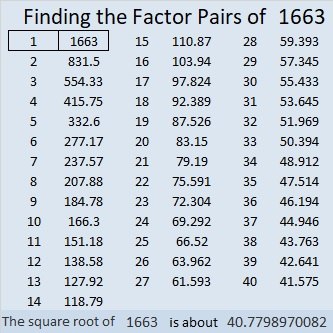# 1663 and Level 1

Contents

### Today’s Puzzle:

Write the numbers 1 to 12 in both the first column and the top row so that those numbers and the given clues function like a multiplication table.### Factors of 1663:

• 1663 is a prime number.
• Prime factorization: 1663 is prime.
• 1663 has no exponents greater than 1 in its prime factorization, so √1663 cannot be simplified.
• The exponent in the prime factorization is 1. Adding one to that exponent we get (1 + 1) = 2. Therefore 1663 has exactly 2 factors.
• The factors of 1663 are outlined with their factor pair partners in the graphic below.How do we know that 1663 is a prime number? If 1663 were not a prime number, then it would be divisible by at least one prime number less than or equal to √1663. Since 1663 cannot be divided evenly by 2, 3, 5, 7, 11, 13, 17, 19, 23, 29, 31, or 37, we know that 1663 is a prime number.

### More About the Number 1663:

1663 is the sum of consecutive numbers in only one way:
831 + 832 = 1663.

1663 is the difference of two squares in only one way:
832² – 831² = 1663.

What do you notice about those two number facts?

This site uses Akismet to reduce spam. Learn how your comment data is processed.